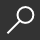Mental Games : Hakoiri Puzzles - 100 Large Print Puzzles
local_shippingFor DeliveryIn Stock.
This item is Non-Returnable.
FREE Shipping for Club Members help### Overview

Hakoiri is a logic puzzle published by Nikoli. A rectangular or square grid is divided into regions. The goal is to place exactly one triangle, one square and one circle in each region. The same figures cannot be placed in adjacent cells, not even diagonally. All the figures must be connected horizontally or vertically.

maths puzzles - mathematical puzzles - math riddles - maths puzzles with answers - math puzzles for adults - maths puzzle games - math brain teasers - simple maths puzzles - maths puzzles with solutions - fun math puzzles - simple maths puzzles with answers - mathematical puzzles with answers - easy maths puzzles - math logic puzzles - fun math problems - math puzzles brain teasers - maths riddles with answers - mathematical riddles - math riddles for adults - math challenge

This item is Non-Returnable

• ISBN-13: 9781718115361
• ISBN-10: 1718115369
• Publisher: Independently Published
• Publish Date: August 2018
• Dimensions: 9.02 x 5.98 x 0.28 inches
• Shipping Weight: 0.4 pounds
• Page Count: 116